# Limits

## Properties of Limits

For many basic functions, the limit at a certain x-value is the function output at that point, as long as the function is defined at that point. However, that rule doesn’t help if you have more complex functions like f(x)=x2 (√ x). For sophisticated functions like this one, you’ll need to use more complex properties of limits.

### Properties of Limits for Simple Functions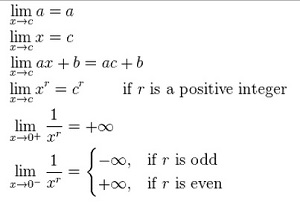### Properties of Limits for General Functions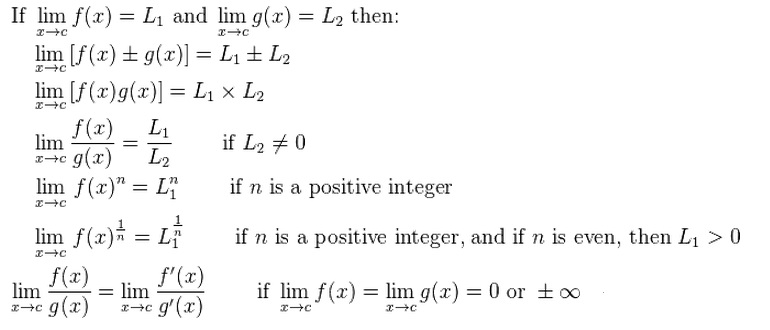### Properties of Limits for Logarithmic and Exponential Functions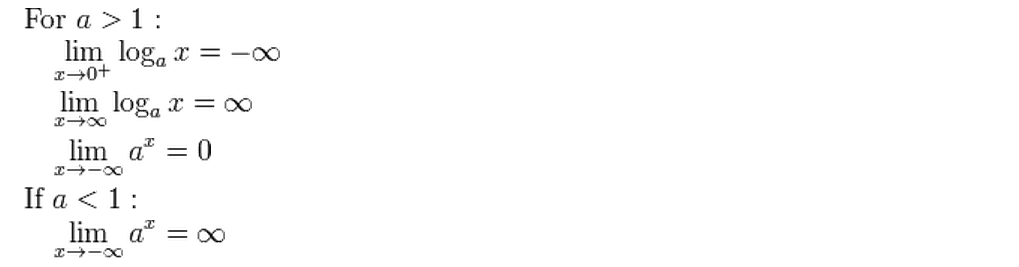### Properties of Limits for Trigonometric Functions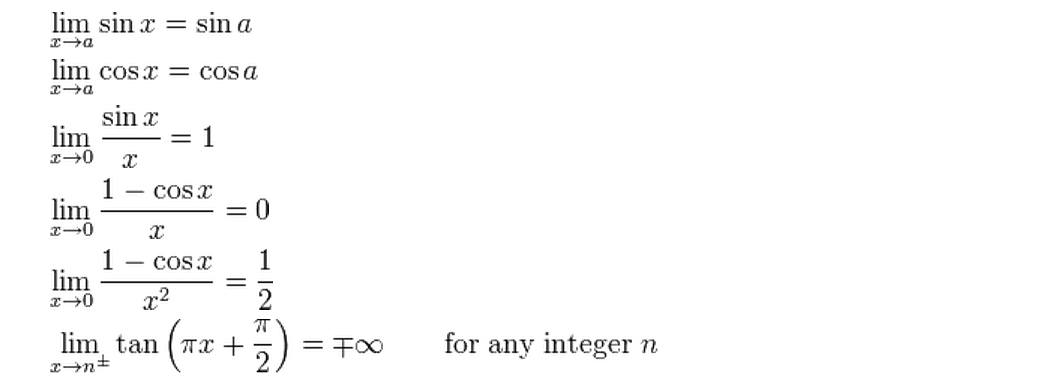The above list is just a few of the many rules for computing limits; they are the most important ones, and the ones you are likely to come across in an introductory calc class.

## Properties of Limits Example

Bear in mind that you must interpret the equations the right way. In other words, a statement like “the limit of a a sum is the sum of the limits” is only true if the two limits on the right hand side (italicized below) of the equation are defined:
Lim x→a (f(x)) + g(x)) = Lim x→a f(x) + lim x→a g(x)

Sample question:Find the limit of f(x) + x2 if lim x-3> f(x) = 17:
Answer: Lim x→3 (f(x) + x2 = 17 + 32 = 17 + 9 = 26.

## Limit Definition in Calculus

The limit is a calculus term meaning the value that a given function will approach as a variable in the function moves towards some number. Its use is extensive in calculus, and has many real world applications. Taking the below function, you would read it as “The limit of a function of x as x approaches y is z.”
lim f(x) = z
x → y

## Squeeze Theorem and the Limit Definition

A simple illustration of the concept is to take the limit of the function y = x + 2 as x approaches 5. In other words, we want to know what value of y the function approaches when x is 5. The variable x will need to approach 5 from both sides, unless otherwise noted (because there are two infinities: negative and positive).

Step 1: Take three steadily nearing values for x from both sides. For example, if you are asked to take the limit of x = 5, you might choose the numbers 4.7, 4.9. 4.99. 5.01, 5.1 and 5.3. Try to choose numbers that are close to x.

Step 2: Solve for x-values. In this example:
When x = 4.7|4.9|4.99| 5 |5.01|5.1|5.3
f(x) = 6.7|6.9|6.99| 7 |7.01|7.1|7.3

Step 3: Note where the value is trending towards. In this example, y is trending towards 7.

This methodology is known as the Squeeze Theorem, or sandwich theorem, which describes the way in which you slowly “squeeze” the value of x towards the intended value. When shown this way, there is an obvious trend towards a value of 7 as x becomes closer to 5 from both directions. Therefore, the limit of the function is 7, which might seem like useless additional work given that simply taking the function at x = 5 gives the same value.

## A more Advanced Illustration of the Limit Definition

To find a case where a limit can offer a solution where simpler mathematics don’t quite work, take the function (x^2-1)/(x-1). Let’s say we want to find the limit at x = 1. If you were to try and make x equal to 1, the function would be undefined due to division by 0. If you repeat the same method followed above, you will get the following results:
When x = 0.7|0.9|0.99| 1 |1.01|1.1|1.3
f(x) = 1.7|1.9|1.990|Undef|2.01|2.1|2.3
The value of f(x) as x approaches 1 becomes closer and closer to 2. This is enough proof for an answer.

## Limits Near Infinity

A typical usage of limits is to find a value for numbers that trend towards infinity. For a function of (4x-2)/(x) there would be an undefined value at x = infinity. If you take arbitrarily increasing numbers, you can try to find a pattern to get the limit of the function as follows:
When x = 10000|1000000 |1000000000
f(x) = 3.9998|3.999998|3.999999998
As x approaches infinity, the value of the function steadily moves towards a value of four.

## Advanced Techniques in Taking Limits

One of the advanced tricks in finding limits approaching infinity is to discard insignificant figures in the equation. In the equation (4x-2)/(x), we can discard the -2, since infinity outweighs -2 substantially. This leads you to 4x/x, which is 4. While not typically enough proof for a limit, the tip can help you tell if your derived limit is plausible or not.

Reference:
Kouba, Duane. “Limits of Functions Using the Squeeze Principle.” http://www.math.ucdavis.edu/~kouba/CalcOneDIRECTORY/squeezedirectory/SqueezePrinciple.html

## How to Find the Slope of a Tangent Line using the Definition of a Limit

There are several ways to find the slope of a tangent line. In calculus, the usual way to find the slope of the tangent line is to take the derivative — because the derivative is equal to the slope of the tangent line at any indicated point. However, if you’re using the slope of the tangent line equation, chances are you haven’t yet covered how to take a derivative yet in your class. The formal definition of the limit can be used to find the slope of the tangent line: If the point P(x0,y<sub<0< sub=””></sub<0<>) is on the curve f, then the tangent line at the point P has a slope given by the formula: Mtan = lim h→0 f(x0 + h) – f(x0)/h.

Sample question: Find the slope of the tangent line to the curve f(x) = 2x2 + 3x – 4 passing through the point P(-1,5).

Step 1: Replace the “x” in your original function by x + h in the first part of the definition of the limit:
mtan = lim h→0 [2 ( x + h ) 2 ] + 3(x + h) + 4]
Step 2: Subtract your original function and divide by h (all you are doing here is completing the definition for the limit):
mtan = lim h→0 [2 ( x + h ) 2 + 3(x + h) – 4] – [2x2 + 3x – 4 ] / h
Step 3: Solve using algebra. The entire purpose of the following steps is to remove the “h” from the denominator to prevent division by zero:

1. FOIL the first part, (x+h)2:
mtan = lim h→0 [2(x2 + 2hx + h2] + 3(x + h) – 4] – [2x2 + 3x – 4 ] / h
2. Multiply out 3(x + h):
mtan = lim h→0 [2(x2 + 2hx + h2] + 3x + 3h – 4] – [2x2 + 3x – 4 ] / h
3. Distribute the negative sign
mtan = lim →0 [2(x2 + 2hx + h2] + 3x + 3h – 4] – 2x2 – 3x + 4 / h
4. Distribute the leading 2:
mtan = lim h→0 [2x2 + 4hx + 2h2 + 3x + 3h – 4 – 2x2 – 3x + 4] / h
5. Cancel out terms (-3x, -2x2, -4)
mtan = lim h→0 [4hx + 3h 2 + 3h ] / h
6. Factor the “h” out:
mtan = lim h→0 h (4x + 2h + 3) / h
= lim h→0 4x + 2h + 3
7. Set the “2h” to zero (because you are taking the limit, “h” is insignificant). In other words, delete it:
= lim h→0 4x + 3

This gives you the function for the slope of the tangent line.
Step 4: Insert your point into the function to find the slope:
mtan = 4 (-1) + 3 = -1
The slope of the tangent line is -1.

That’s it!

## How to Figure out When the Limit Does Not Exist

As long as the function’s values approach the same value from both sides, then the limit exists — even if the function’s value at that point is undefined. For example, if a function is undefined at x = 3, test both sides (2.999 and 3.001) to find the limit. The formal definition of a limit states that the limit must be a singular value. When a particular function seems to approach multiple values, the limit does not exist. In most cases, you’ll be able to determine if the limit does not exist if you attempt to find the limit of a function using a graph.

Step 1:Look at the graph. If the graph is going in completely different directions at the particular value you are looking at, the limit most likely does not exist. You can test this if you find the limit of a function using a graphing calculator, because you’ll be able to zoom in on smaller and smaller increments to test the behavior of the graph.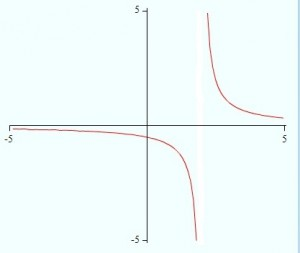Step 2: Find the limit of the function numerically by building a table with small increments either side of the function’s value. If the table shows a trend to different numbers either side of the value, then the limit does not exist.

Step 3:Use your algebra skills to look at the behavior of a function. For example, the limit of the function (-1/(1-x)-cos(x))/(2cos(x)sin(x)) does not exist at x = 0. As the function approaches zero, the numerator approaches -2 and the denominator approaches 0. Using this logic, you can determine that the limit from the left is negative infinity and the limit from the right is infinity.

Tip: Technically, a limit doesn’t exist if the value at that function is infinity. But knowing that a number approaches infinity at a certain point is extremely useful, so we say that: lim f(x) = ∞.

## How to Find a Limit in Calculus using the Squeeze Theorem

The squeeze theorem uses a table of values to get closer and closer to — or “squeeze close to” the limit. Put simply, the Squeeze Theorem uses values steadily approaching c from above and below it to indicate the limit of the function.

Sample question: Find the limit of x, as x approaches 0.
Step 1: Set up an equation (you can skip this step if you want to, but many calculus professors will require you include it).
f(x) = x
c = 0
lim f(x) = lim x = L
x→c x→0
Step 2: Set up a small table to utilize the Squeeze Theorem. Use values that approach c significantly in order to form an accurate limit. Using -1000, -500, and -100 might be approaching a c of 0, but they are too broad to give an exact picture.

 x -0.30 -0.10 -0.01 -c=0 0.01 0.10 0.30 f(x)

Step 3: Solve for f(x) at the values of x→c that you set up. You can also solve for f(x) at c, but keep in mind that the value at x = c is not always indicative of the limit in more complex functions, such as 1/x.

 x -0.3 -0.1 -0.01 -c=0 0.01 0.1 0.3 f(x) -0.3 -0.1 -0.01 0 0.01 0.1 0.3

Step 5: Rewrite the limit function properly, and give the limit.
lim f(x) = lim x = L = 0
x→0 x→0

Tips: One common analogy for the Squeeze Theorem is “the two policemen and a drunk” story: If two policemen are on either side of a drunk, and escort him to a cell, the drunk will end up in the cell. While not an exact correlation to the Squeeze Theorem, it can be used to help remember it.

## Solve Limits Step by Step Using The Formal Definition of a Limit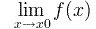This section shows you how to solve limits using the formal limit definition.
The formal definition of a limit is:
“For any ε > 0, there is a δ > 0 so that |f(x)-L| < ε when 0 < |x-a| < δ”.
What it means is that when x gets close to a number, f(x) gets close to L, a limit. If you want to find limits, it’s more intuitive to solve limits numerically or solve limits graphically. However, it is possible to solve limits step by step using the formal definition.

## Solve Limits Step by Step Example

Sample question: Prove that the limit of f(x) = 2x + 4 is 10 as x approaches 3.

Step 1: Assign δ and ε to your x-value and your function, setting them up as inequalities:
0 < |x-3| < δ
|(2x+4)-10| < ε

Step 2: Find a value for δ (in this example, δ is |x-3|) in terms of ε. Start with the ε portion from step 1:
|(2x+4)-10| < ε =
|(2x-6| < ε = (Simplifying)
2|x-3| < ε =
|x-3|< ε/2 =
δ< ε/2

Step 3: Turn the inequality into an equals sign:
δ= ε/2

Step 4: Use algebra to manipulate the δ from Step 1. This time, your goal is to go from 0 < |x – 3|< δ): to |(2x + 4) – 10| < ε
0 < |x – 3|< δ =
0 < |x – 3|< ε / 2 = (using the value for delta you derived in Step 2)
0 < 2|x – 3|< ε =
0 < |2x – 6| < ε =
0 <|2x + 4|- 10 < ε

Tip: Step 2 is really all guesswork. Look for ways to make the numbers in the ε portion of the equation equal the δ numbers. In this example, there is a “3” in δ, so we simplified to 2x-6 because 6/2=3. In Step 4, the sequence is essentially reversed to prove the formula works.

## Finding Limits with Direct Substitution

Probably the most intuitive way to find a limit is to evaluate a limit graphically or numerically (through a table), using the squeeze theorem. However, in some cases it’s actually easier—and faster—to find a limit with direct substitution. If you’ve ever put a specific value into an equation in algebra (like putting x = 2 into the function y=x+10), then you have already performed direct substitution. It’s really that easy!

Direct substitution works with basic functions, like constant functions or linear functions. Constant functions are functions which are constant and do not vary. For example, f(x) = 10, f(x) = 6 and f(x) = 99.99 are all constant functions. Linear functions yield straight lines in a graph; they have “x” as the input variable and the “x” has an exponent of one. Examples of linear functions are f(x) = x, f(x) = 2x – 2 and f(x) = x + 1.

## Find limit direct substitution: Steps

Sample problem: Find the limit of f(x) = 9x – 2 at x = 6.

Step 1: Identify the function as a constant or linear function. The function is a linear function.

Step 2: Substitute the “x” value (the point at which you want to find the limit) into the function.

f(x) = 9x – 2
f(x) = 9(6) – 2
f(x) = 54 – 2
f(x) = 52

The limit of f(x) = 9x – 2 as x approaches 6 is 52.

Tip: Direct substitution can also work for polynomials and radicals, as long as you are sure the function is defined at the x-value you want to find the limit at. For example, you can use direct substitution for all values of f(x) = 1/x, except at 0 (because division by zero is undefined). If your algebra isn’t strong, a good rule of thumb is that you shouldn’t use direct substitution to solve for the limit unless you are sure that the function is defined for all real numbers.

## How to Find the Limit of a Function Graphically

It’s almost impossible to find the limit of a function without using a graphing calculator, because limits aren’t always apparent until you get very, very close to the x-value. The TI-89 is one of the best calculators for calculus; if you have another brand (like an HP), the steps will still be the same, but you’ll have to refer to your calculator’s user guide for the specific key strokes.

Sample problem: Find the limit for the function 3x2 – 3 / x2 – 9 as x approaches 0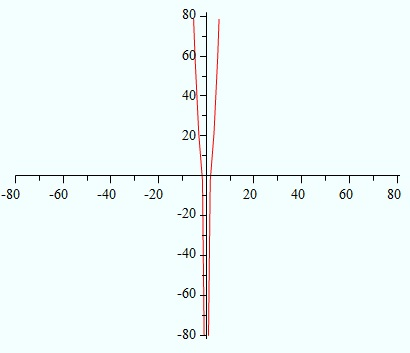Step 1: Enter the function into the y1 slot of the “Y=” window. To open the window, press the Diamond key, then press the F1 key. Type the equation in and then press enter.

Step 2: Press the Diamond key, then F2 to enter the Window screen. This screen allows you to tell the calculator what view you want of the graph.

Step 3: Type a value into the xmin, xmax, ymin and ymax areas. For many functions, this will be guesswork until you can determine the behavior of the graph. For this particular example, type -5 for xmin, 5 for xmax, -1000 for ymin and 1000 for ymax (we are choosing a small window for the x-values because we want to see the limit around x = 0).

Step 4: Press the Diamond key and then F3 to graph the function.

Step 5: Press F3 for Trace.

Step 6: Press 0 and then press ENTER to see the y-value for x = 0. In this case, it will return nothing—x is undefined at this point.

Step 7: Repeat step 6, entering smaller increments close to x, like .001, .0001 and .00001. You should see the function values in this particular case approaching infinity.

Tip: Typically, your xmin and xmax are very small and your ymin and ymax are very large. If you are having trouble viewing the graph, construct a table of values so that you have a better idea of the graph’s behavior.

## How to Find the Limit of a Function Numerically

The limit of a function is a particular value of x that the function approaches. You can find the limit of a function by graphing it—then the limit usually becomes obvious. But what if you have a function whose graph doesn’t help you? That sometimes happens when the limit is undefined at one particular point (you won’t be able to see it at all on a graph—it will appear to be a continuous function) or if the graph keeps on going towards infinity (“infinity” isn’t an x-value you can graph!). To find the limit for these functions, you’ll want to find the limit of a function numerically, using a table of values. You could make a table of values by hand. However, for most of the functions you’ll be dealing with in calculus, making a table of values by hand is impractical. The solution is to use your TI-89 graphing calculator.

Sample question. What is the limit of 3x2 – 3 / x2 – 9 as x approaches 0?

Step 1: Enter the function into the y1 slot of the “Y=” window. To open the window, press the key, then press the key. Type the equation in and then press enter.
Step 2: Set the table options. To set the table options, press the and then press . Change the settings for the given limit. For this example, set the table to start at -1 (just before the limit) and set the increments (∆) to 1.
Step 3: Open the table: press the and then press . Note that the table states “undef” for x = 0 and -9 for x = -1. -9 could be the limit, but to be sure, look at smaller increments.
Step 4: Change the table options to smaller increments. For this example, use increments of .1, using the procedure in step 2. Note the limit now looks like it might be -309.
Step 5: Continue changing the table increments to smaller and smaller values like hundredths, thousandths or more. When the numbers starts to stabilize around a point, you’ll know the limit. For this particular function, the numbers keep on increasing towards infinity.

Tips:

1. As you decrease the increments, you’ll also want to change the place where your table starts (move the table to start at a place close to the x-value you are looking at). Otherwise, you’ll find yourself paging through hundreds of table values.
2. If you want to check values very close to a number, you can also ask for individual table entries instead of the calculator returning a long list of incremental values. From the table options screen, arrow down to “Independent” and change the input from “Auto” to “Ask.” View the table, press ENTER and then enter an x-value (like -0.000000001).

L’Hospital’s Rule

Limits are used in calculus to find derivatives. But sometimes, limits don’t work when the limits are indeterminate. Examples of indeterminate limits are 00, or -∞-∞. When you encounter indeterminate limits you use L’Hospital’s rule to find limits using derivatives. The formal definition of L’Hospital’s rule looks a little ugly: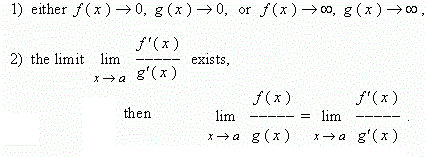However, all it’s really saying is that in special cases you can use the limit of the derivative to find the limit of the function.

## L’Hospital’s Rule: Sample Problem

Sample problem: Use L’Hospital’s rule to find the limit as x approaches zero for the function sin(x)x :

Step 1: Take the limit of the function to make sure you have an indeterminate form.
lim x→0 sin(x)x = 00
If you don’t have an indeterminate form of the limit (i.e. if the numerator and the denominator in the fraction are both zero or infinity), you don’t need L’Hospital’s rule.

Step 2: Identify f(x) and g(x) from your function. The top function is f(x) and the bottom function is g(x). In this case, f(x) is sin(x) and g(x) is x.

Step 3: Find the derivative for f(x) and g(x):
f'(sin x) = cos x
f'(x) = 1

Step 4: Take the limit of the derivative functions from Step 3:
lim x->0 (cos x) = 1
lim x->0 (x) = 1
Therefore, the limit of the function cos(x)x = 1/1.

L’Hospital’s rule states that the limit of this derivative function is also the limit of the function itself, so:
lim →0 sin(x)x = 1

That’s it!

## Limits for Polynomial Functions

There’s more than one way to skin a cat, and there are multiple ways to find a limit for polynomial functions or radical functions. This can be extremely confusing if you’re new to calculus. But the good news is — if one way doesn’t make sense to you (say, numerically), you can usually try another way (i.e. graphically).

You can find a limit for polynomial functions or radical functions in three main ways:

Graphical and numerical methods work for all types of functions; Click on the above links for a general overview of using those methods. You can use those methods to find limits for polynomial functions or radical functions that are very simple. If you have a simple polynomial function or radical function you might also be able to use direct substitution to find limits. However, you can’t use direct substitution if you have a complicated function (like f(x) + g(x)).

This article walks you through finding limits algebraically using Properties of limits . Properties of limits are short cuts to finding limits. They give you rules — very specific ways to find a limit for a more complicated function. For example, you can find limits for functions that are added, subtracted, multiplied or divided together.

## Limit for Polynomial functions or Radical Functions: Steps

Sample problem: What is the limit at x = 2 for the function
f(x) = (x2 +√2x)?

Step 1: Look at the Properties of Limits rules and identify the rule that is related to the type of function you have. The function above is a combination of a polynomial function ((x2) and a radical function ( √ 2x). It’s what we call an additive function, f(x) + g(x), so the rule that applies (found in the properties of limits list) is:
lim x→a [ f(x) ± g(x) ] = lim1 ± lim2

Step 2: Insert your function into the rule you identified in Step 1.
lim x→2 [ (x2 + √ 2x) ] = lim x→2 (x2) + lim x→2(√ 2x).

Step 3: Evaluate the limits for the parts of the function. If you’ve broken your function into parts, in most cases you can find the limit with direct substitution:
lim x→2 [ (x2 + √ 2x) ] = (22 + √ 2(2)) = 4 + 2

Step 4: Perform the addition (or subtraction, or whatever the rule indicates):
lim x→2 [ (x2 + √ 2x) ] = 4 + 2 = 6
That’s it!

## How to Find the Limit of Linear Functions

Linear functions are functions that have a graph that consists only of a single straight line. Line functions typically adhere to the form of y = mx + b. In this form, m represents the slope of the function, and b is where the line intersects the y-axis. This form is also known as the slope-intercept form. Slightly different steps are needed to solve for limits of linear functions approaching values other than infinity, and to solve for limits of linear functions at infinity.
Note: If you are uncomfortable with algebra or the formal definition of a limit, you can use a Squeeze Theorem table to find limits of linear functions that don’t approach infinity. If you’re trying to find limits of linear functions at infinity, you can also use a slightly modified version of the Squeeze Theorem.

### Solving for limits of linear functions approaching values other than infinity.

Sample problem: Find the limit of 2x+2 as x tends to 0.
Step 1: Set up an equation for the problem:Use the normal form for a limit, with c equal to 0, and f(x) equal to 2x+2.
f(x) = 2x+2
c = 0
lim f(x) = L = lim 2x+2
x → c x → 0
Step 2: Solve for the limit of the function, using some basic properties of linear functions:

1. The limit of ax as x tends to c is equal to ac
2. The limit of a as x tends to c is a
3. The limit of a + b is equal to the limit of a plus the limit of b

Using this logic, the limit is 2 as x approaches 0.
lim 2x+2 = lim 2x + lim 2 = 0 + 2 = 2
x → 0 x → 0 x → 0

### Solving for limits of linear functions approaching infinity.

Sample problem: Find the limit of 2x+2 as x tends to 0.
Step 1: Repeat the steps as above, but this time solve for the limit as x approaches infinity.
f(x) = 2x + 2
c = ∞
lim 2x + 2 = lim 2x + lim 2 = ∞ = Limit does not exist
x → ∞ x → ∞ x → ∞

Tip: Since the limit goes to infinity when you times infinity by 2, the limit of the function does not exist due to infinity not being a real number. There is one special case where a limit of a linear function can have its limit at infinity taken: y = 0x + b. Since the 0 negates the infinity, the line has a constant limit. This would appear as a horizontal line on the graph.

## How to Find the Limit of a Secant Function

Finding the limit of a secant function can seem imposing when you look at a graph of the function, but following the rules for finding limits using the Squeeze Theorem makes it surprisingly simple. One key fact to keep in mind is that if a limit does not approach the same value from the left and the right, then the limit does not exist.

Sample Problem: Find the limit of sec (x) as x approaches 1.

Step 1: Set up your equation L represents the limit, f(x) is the function you are finding the limit of, and c is the value x is approaching for determining the limit.
f(x) = sec(x)
c = 1
lim f(x) = lim sec(x) = L
x→c x→1
Step 2: Design a table to use the Squeeze Theorem to evaluate the limit. You can include the c value in the table, but remember that the value of the function at c is inconsequential to the limit.

 x 0.70 0.90 0.99 c=1.00 1.01 1.10 1.30 f(x)

Step 3: Evaluate for the value of the function at the x values you set up, using a calculator.

 x 0.7 0.9 0.99 c=1.00 1.01 1.1 1.3 f(x) 1.00007 1.00012 1.00015 1.00015 1.00016 1.00018 1.0026

Step 4: Analyze the calculated values to find the limit. If the limit is not clear, but the values do seem to be heading towards a limit from both sides, continue to use numbers closer and closer to c to narrow down the limit. In this case the values are trending towards 1.00015. Rewrite the equation to give your answer.
lim f(x) = lim sec(x) = L = 1.00015
x→c x→1

Sample ProblemFind the limit of sec (x) as x approaches 90.

Step 1: Set up your function.
lim f(x) = lim sec(x) = L
x→c x→90
Step 2:Repeat Steps 2 and 3 above for the new values.

 x 89.7 89.7 89.99 c=0.90 90.01 90.1 90.3 f(x) 190.99 572.96 5729.5 Undefined -5729.5 -572.96 -190.99

As you can see, the function is undefined at 90, and approaching 90 from the left tends towards infinity, while approaching 90 from the right tends towards negative infinity. In this case, the limit of a secant does not exist, and stating such qualifies as the correct answer. For the secant function, this will occur at 90 and at every interval of 180 either direction from it.

## How to find the upper limit of an integral

One of the main topics in calculus is understanding how to calculate integrals, whether they are of indefinite or definite form. The main difference between the two is that an indefinite integral has no bounds while the definite integral has two calculus limits: a lower and upper bound. When you solve for the upper limit of an integral, you’re solving for a definite integral with an upper bound.

## How to find the upper limit of an integral in calculus: Steps

Step 1: Identify the function in question. In an integral, this is the value in between the integral symbol and the integration constant (usually denoted as ‘dx’ or perhaps ‘dy’). As an example, we’ll name the function to be something simple such as ‘f(x) = 4x’.
Step 2: Identify the calculus limits of the integral. These values are typically denoted at the top and bottom of the integral sign. The upper bound is the value up top and the lower bound is the value at the bottom of the symbol. We’ll allow the upper bound to be 2 while the lower bound is 1.
Step 3: Perform the integration of the function using indefinite integral rules. For f(x)=4x, raise the power of the variable by one and divide the entire function by the new exponent of the variable. For example, the integral of f(x) = 4x becomes 2x2.
Step 4: Insert the upper bound of the integral into the newly integrated function. We replace our variable with the previously identified upper bound (which we valued at 2). Our integrated function then becomes f(x) = 2(2)2, where the value in the parentheses is the upper bound that replaced the variable.
Step 5: Perform the mathematical computation. Do not forget the order of operations – your answer could result in something radically different if you fail to follow this step!
f(x) = 2(2)2 = 2(4) = 8. So for the function f(x) = 4x, integrating it with an upper bound of 2 will result in the value 8.

That’s it!

## How to Find Limit of Sums: Upper and Lower

Upper and lower sums enable you to find the area under a curve. A lower sum is calculated by adding up the areas of inscribed rectangles, while an upper sum is calculated by adding up the areas of circumscribed rectangles.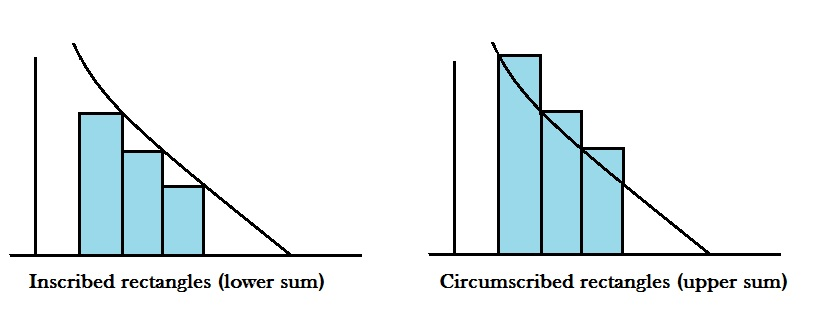When the number of rectangles increases to infinity, the upper and lower sums converge on one number: the limit.

## Find Limit of Sums Algebraically

If you want to find limits of upper and lower sums, it’s incredibly tedious to perform the process algebraicially. Several pages of algebra are required to solve for the upper and lower sums of even the simplest polynomial — like y=3x2. if you want to use algebra, follow the (very lengthy) explanation that Stan Brown provides on his article “Area by Upper and Lower Sums.” You can find that article HERE.

## Find Limit of Sums with the Fundamental Theorem of Calculus

Fortunately, there’s an easier way to find the limit by hand: By using the fundamental theorem of calculus. The fundamental theorem allows you to evaluate definite integrals for functions that have indefinite integrals. The first part of the fundamental theorem states that if you are evaluating indefinite integrals between two points, all you have to do is subtract the value of the integral at the first point from the value of the integral at the second point. If it sounds complicated, it’s actually a cake walk compared to trying to use algebra to find limit of sums! The second part of the fundamental theorem of calculus gives you the indefinite integral for a function.

## Find Limit of Sums on the TI 89

A third option to find limit of sums is the TI89 using the LIMIT command. The LIMIT Command TI 89 guides you through the process, step-by-step.